# Electronics and Communication Engineering - Digital Electronics

46.

A 3 bit up-down counter can count from

 A. 000 to 111 B. 111 to 000 C. 000 to 111 and also from 111 to 000 D. none of the above

Explanation:

Since it is an up-down counter it can count upwards or downwards.

47.

The density of dynamic RAM is

 A. the same as static RAM B. less than that of static RAM C. more than that of static RAM D. either equal or less than that of static RAM

Explanation:

Dynamic RAM has higher packing density and requires lesser space.

48.

The gates G1 and G2 in the figure have propagation delays of 10 n sec. and 20 n sec. respectively. If the input Vi makes an output change from logic 0 to 1 at time t = t0 then the output wavefrom V0 is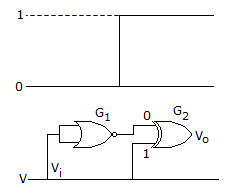A.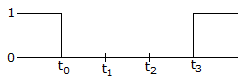B.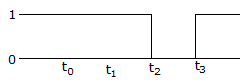C.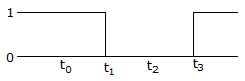D.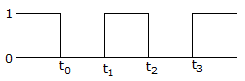Explanation:

Truth table are as follows.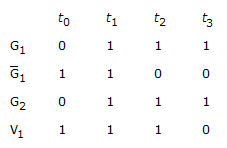49.

A 3 input NAND gate is to be used as inverter. Which of the following will give better results?

 A. The two unused inputs are left open B. The two unused inputs are connected to 0 C. The two unused inputs are connected to 1 D. None of the above

Explanation:

Unused inputs should be given high input.

50.

The maximum counting range of a four stage counter using IC 74193 is

 A. 0 to 1023 B. 0 to 4093 C. 0 to 65535 D. 0 to 131071

Explanation:

IC 74193 is a divide by 16 counter.

Since 4 stages are used, counting range = 164 = 65536.